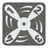Repeating Decimals
Elementary+
English

In mathematics, a repeating decimal is a way of representing a rational number. A decimal representation of a number is called a repeating decimal if at some point there is some finite sequence of digits that is repeated infinitely. For example: the decimal representation of 1/3 = 0.3333333… or 0.(3) becomes periodic just after the decimal point, repeating the single-digit sequence "3" infinitely. ... .

Input: Two arguments. A numerator and a denominator as integers.

Output: The decimal representation of the fraction in the bracket format as a string.

Example:

```convert(1, 3) == "0.(3)"
convert(5, 3) == "1.(6)"
convert(3, 8) == "0.375"
convert(7, 11) == "0.(63)"
convert(29, 12) == "2.41(6)"
convert(11, 7) == "1.(571428)"
convert(0, 117) == "0."
convert(4, 2) == "2."
```

How it is used: This is the important part for mathematical software. And of you need to help your children with homework.

Precondition:
0 ≤ numerator ≤ 1000
1 ≤ denominator ≤ 1000

You should be an authorized user in order to see the full description and start solving this mission.
Settings
Code:
CheckiO Extensions

CheckiO Extensions allow you to use local files to solve missions. More info in a blog post.

In order to install CheckiO client you'll need installed Python (version at least 3.8)

Install CheckiO Client first:

`pip3 install checkio_client`

`checkio --domain=py config --key=`

Sync solutions into your local folder

`checkio sync`

(in beta testing) Launch local server so your browser can use it and sync solution between local file end extension on the fly. (doesn't work for safari)

`checkio serv -d`

Alternatevly, you can install Chrome extension or FF addon

`checkio install-plugin`
`checkio install-plugin --ff`
`checkio install-plugin --chromium`

Read more here about other functionality that the checkio client provides. Feel free to submit an issue in case of any difficulties.

Sync...